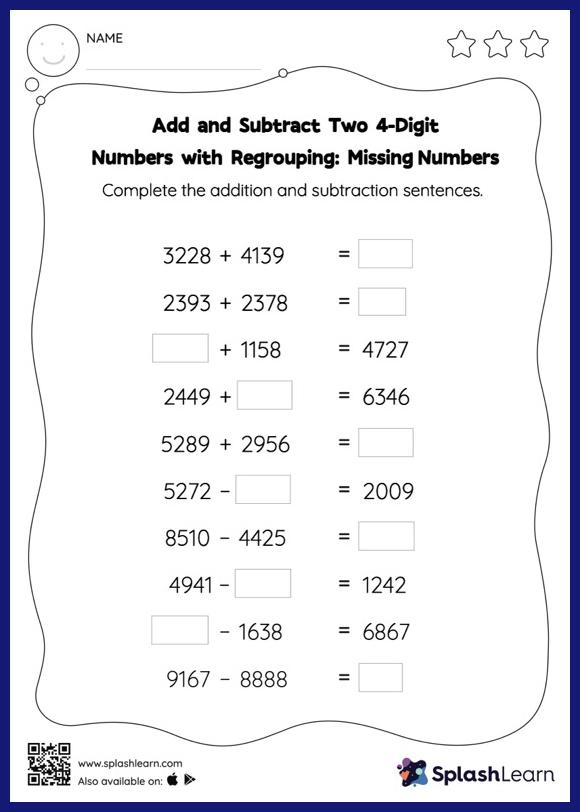# Add and Subtract Two 4-Digit Numbers with Regrouping: Missing Numbers Worksheet

Home > Add and Subtract Two 4-Digit Numbers with Regrouping: Missing NumbersIn this worksheet, students must find the missing number using the relationship between addition and subtraction. To solve add and subtract two 4-digit numbers with regrouping worksheet, they must also regroup the numbers. As the worksheet uses a horizontal format, it allows for more creativity when it comes to how the student solves the problem. In contrast, in the vertical format, students usually employ the standard method to solve.